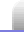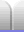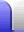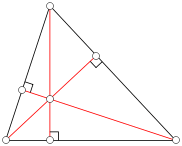# Triangle Centres

### IncentreWe bisect an angle if we draw a line through it that cuts the angle exactly in half. The resulting line is sometimes called a bisector.

If we bisect all three angles of a triangle the bisectors will intersect at a single point called the incentre. The incentre is the centre of the incircle – a circle that will just fit within the triangle. The sides of the triangle are tangents to the circle.

Why it works: By bisecting the angles we get lines that are equidistant from the sides. The incentre is thus equidistant from all three sides of the triangle. The perpendicular distance to the sides is the radius of the incircle.

### CircumcentreWe bisect a line if we draw a perpendicular line through the line's midpoint.

If we bisect all three sides of a triangle the bisectors will intersect at a single point called the circumcentre. The circumcentre is the centre of the circumcircle – a circle that just touches the three points of the triangle.

• The circumcentre of an acute angle triangle is inside the triangle.
• The circumcentre of a right angle triangle is on the hypotenuse.
• The circumcentre of an obtuse angle triangle is outside the triangle.

Why it works: By bisecting the sides of the triangle we get lines that are equidistant from the points at each end of the line – the points of the triangle. The circumcentre is thus equidistant from all three corners of the triangle, so the distance to the points is the radius of the circumcircle.

Want more sides? All triangles have a circumcentre but not all polygons with more sides do. For example, not all quadrilaterals fit inside a circle with each corner touching the circle. Those that do are called cyclic quadrilaterals. They have the interesting feature that opposite internal angles add up to 180°.

### CentroidIf we take the midpoints of each side (as found when locating the circumcentre) and connect each of those three points with the opposite triangle corner, the resulting median lines intersect at a single point called the centroid.

The centroid is the centre of mass of the triangle; cut out a triangle and it will balance on a pin at its centroid.

Why it works: Each median divides the area of the triangle in half. The centroid thus has an equal area – and hence equal mass – of the triangle on every side of it.

### OrthocentreThe orthocentre is point is found by taking a perpendicular line through each side, that passes through the opposite corner.

In other words, the orthocentre is the intersection of the three heights (or altitudes) of the triangle.

The triangle drawn joining the base points of the three altitudes has the smallest perimeter of any triangle inscribed in the original triangle.Home Astronomy Chemistry Electronics & Computers Mathematics Physics Field Trips Turn on javascript for email link